# Surfaces, Theory of

The following article is from The Great Soviet Encyclopedia (1979). It might be outdated or ideologically biased.

## Surfaces, Theory of

the branch of differential geometry that studies the properties of surfaces. The classical theory of surfaces considers properties that are invariant under motions. One of the main tasks of the classical theory of surfaces is that of making measurements on a surface. The aggregate of facts obtained by measurements on a surface constitutes the intrinsic geometry of the surface. Such concepts as the length of a curve, the angle between two directions, the area of a region, geodesies, and the geodesic curvature of a curve pertain to the intrinsic geometry of the surface.

The intrinsic geometry is determined by the first fundamental form of the surface

(1) ds2 = E du2 + 2F du dv + G dv2

Here E. = ru2, F = ru rv,,., and G = rv2,., where r = r(u, v) is the radius vector of a variable point on the surface and u and v are the curvilinear coordinates of the point. The first fundamental form expresses the square of the differential of an arc of a curve on the surface. Thus, if we know the functions E. = E(u, v), F = F(u, v), and G = G(u, v) and the intrinsic equations u = u(t) and v = v(t) of the curve, we can determine the length of the curve by integrating ds. Moreover, there exist formulas that, for given E, F, and G, express the angle between two curves and the area of a region in terms of the intrinsic equations of these curves and the intrinsic equation of the contour of the region.

The spatial structure of the neighborhood of a point on the surface is studied by means of the second fundamental form of the surface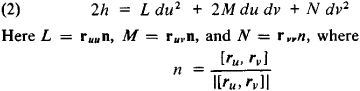is the unit vector of the normal to the surface. Apart from higher order terms in du and dv, the quantity A is equal to the distance from the point of the surface with coordinates u + du and v +f dv to the tangent plane y at point M with coordinates u and v, and a plus or minus sign is selected for the distance according to the side of γ on which point is located. If form (2) is of fixed sign, then the surface in a sufficiently small neighborhood of M lies on one side of γ and Λ/is called an elliptic point (Figure 1). If form (2) changes sign, then the surface in the neighborhood of M lies on both sides of γ, and M is said to be hyperbolic (Figure 2). If form (2) is of fixed sign but can equal zero when du and dv are not simultaneously equal to zero, then M is said to be a parabolic point. An example of the structure of a surface in the neighborhood of a parabolic point is given in Figure 3.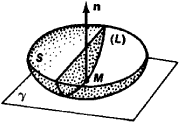Figure 1

A more exact characterization of the spatial form of a surface can be obtained by investigating the geometric properties of curves on the surface. Let M be some point of surface S, and let η be the unit vector of the normal to the surface at M. The curve L of intersection of S with a plane passing through η in the direction du/dv is called the normal section in this direction. The curvature of the normal section is called the normal curvature 1/R, which is calculated by the formula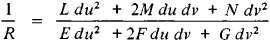The normal curvature of a surface at a given point M in a given direction du/dv may be considered as a measure of the curvature of the surface at M in the direction du/dv. The maximum and minimum values of the normal curvature at a given point are called the principal curvatures, and the corresponding directions on the surface are called the principal directions. The relationship between the curvature of an arbitrary normal section at a given point and the principal curvatures is a simple one and is given by the equation of Euler. If the principal curvatures at M are different, then two different principal directions exist at this point. Curves whose directions at each point are principal are called lines of curvature. Directions in which the normal curvature is equal to zero are called asymptotic directions, and curves that have an asymptotic direction at each point are called asymptotic curves. A surface consisting of elliptic points, such as a sphere, has no asymptotic curves. A surface consisting of hyperbolic points has two families of asymptotic curves, for example, the two systems of rectilinear generatrices of a hyperboloid of one sheet. A surface consisting of parabolic points has one system of asymptotic lines— a system of rectilinear generators. Further study of the properties of arbitrary curves on a surface (chiefly the curvature of curves) is closely associated with the curvatures of the normal sections. It follows from Meusnier’s theorem that the curvature k at a given point M of an arbitrary curve Γ is given by the equation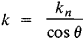where kn is the curvature of the normal section L at M in the direction of the tangent to Γ, and θ is the angle between the principal normals to Γ and L at this point.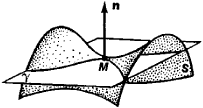Figure 2

Surfaces are said to be isometric if they admit a one-to-one correspondence such that the lengths of corresponding curves are equal. Isometric surfaces have an identical intrinsic geometry. Their spatial structure, however, may differ, and the principal curvatures at corresponding points may also be different. For example, the neighborhood of a point on a plane is isometric to some neighborhood of a point on a cylinder but has a different spatial structure. According to Gauss’ theorem (1826), however, the product K of the principal curvatures 1/R1 and 1/R2 at M does not change under isometric transformations of the surface and provides an intrinsic measure of the curvature of the surface at M. The quantity K is called the total, or Gaussian, curvature of the surface at M and is given by the expression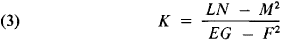which is called Gauss’ equation. It should be noted that, in accordance with Gauss’ theorem, the total curvature can be expressed in terms of the coefficients of the first fundamental form and their derivatives alone. The classification of the points of a regular surface given above can be associated with the values of the total curvature: the curvature is positive at an elliptic point, negative at a hyperbolic point, and zero at a parabolic point.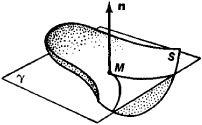Figure 3

Another measure of the curvature of a surface is the mean curvature, which is equal to half the sum of the principal curvatures of the surface and is considered in many problems of the theory of surfaces. For example, minimal surfaces, whose mean curvature at each point is equal to zero, are one of the entities investigated by the theory of surfaces.

The question of whether a given surface is bendable is of great importance in the theory of surfaces. Mathematically this question can be stated as follows: Is it possible to incorporate a given regular surface into a one-parameter family of isometric noncon-gruent regular surfaces (congruent surfaces are surfaces that can be made to coincide by a motion)? Sufficiently small pieces of surfaces of positive and negative curvature permit of continuous bendings. The Soviet geometer N. V. Efimov established that there exist surfaces with a flatpoint— that is, a point where all normal curvatures are equal to zero—such that an arbitrarily small neighborhood of the point does not permit of bending. The theory of surfaces deals not only with the possibility of bending but also with bendings of special types.

The problem of the bending of surfaces is closely related to that of determining a surface from given fundamental forms, a problem that received a complete solution in the work of several mathematicians: the German K. Gauss, the Russian K. M. Peterson, the Italians G. Mainardi and D. Codazzi, and the Frenchman O. Bonnet. Since the value of the total curvature K of a surface can be expressed in terms of the coefficients of the first fundamental form, equation (3) is one of the equations that connect the coefficients of the first (1) and second (2) forms. In 1853, Peterson established two more such equations: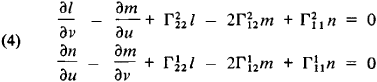where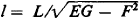,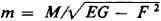, and n =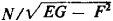and where the Γijk are Christoffel symbols of the second kind. A kind of converse assertion is also valid: if one of two forms is positive definite and the coefficients of the forms satisfy equations (3) and (4), then there exists a surface, determined up to position, for which these forms will be the first and second fundamental forms.

One of the most important problems of the theory of surfaces is that of finding tests that permit us to determine from the two fundamental quadratic forms of a surface (in arbitrary coordinates) whether or not the surface belongs to a given class of surfaces. The methods of tensor calculus are used to solve this general problem as well as many other problems of the theory of surfaces.

In the early 20th century a new trend appeared in the theory of surfaces: the investigation of a surface in the large on the basis of given properties of the neighborhoods of the surface’s points. For example, L. G. Shnirel’man and L. A. Liusternik proved the existence of three closed geodesies on regular closed surfaces homeomorphic to a sphere. The extension of smooth surfaces sometimes results in the appearance of singular points on the surfaces. Thus, when a noncylindrical developable surface is extended, it reaches an edge (which reduces to a point in the case of a cone). The consideration of surfaces over their entire extent and of surfaces with singular points—that is, the rejection of the requirements of differentiability— necessitated the invention of fundamentally new methods of investigation and the use of methods from other branches of mathematics. The development of the theory of surfaces in this direction led to the creation of important branches of geometry. For example, profound and fundamentally new results were obtained by A. D. Aleksandrov and A. V. Pogorelov in the theory of convex surfaces. Aleksandrov proposed a new method for investigating convex surfaces that is based on the approximation of convex surfaces by convex polyhedrons.

The properties of surfaces considered above do not change under isometric mappings of the entire space—that is, they belong to the metric theory of surfaces. Surface properties that are invariant with respect to some other group of mappings of space, such as the group of affine or projective transformations, also are studied in the theory of surfaces. The affine theory of surfaces considers properties that are invariant under equiaffine transformations, or affine mappings that preserve volume. The projective theory of surfaces considers properties that are invariant under projection.

### REFERENCE

Rashevskii, P. K. Kurs differentsial’noi geometrii, 4th ed. Moscow, 1956.
Norden, A. P. Teoriia poverkhnostei. Moscow, 1956.
Pogorelov, A. V. Differentsial’naia geometriia, 5th ed. Moscow, 1969.
Kagan, V. F. Osnovy teorii poverkhnostei v tenzornom izlozhenii, parts 1–2. Moscow-Leningrad, 1947–48.
Blaschke, W. Differentsial’naia geometriia igeometricheskie osnovy teorii otnositel’nosti Einshteina, vol. 1. Moscow-Leningrad, 1935. (Translated from German.)
Aleksandrov, A. D. Vnutrenniaia geometriia vypuklykh poverkhnostei. Moscow-Leningrad. 1948.
Pogorelov. A. V. Vneshniaia geometriia vypuklykh poverkhnostei. Moscow, 1969.
Finikov, S. P. Proektivno-differentsial’naia geometriia. Moscow-Leningrad, 1937.
Shirokov, P. A., and A. P. Shirokov. Affinnaia differentsial’naia geometriia. Moscow, 1959.
Blaschke, W. Vorlesungen iiber Differentialgeometrie, vol. 2. Berlin, 1923.
Bianchi, L. Lezioni di geometria differenziale, 3rd ed. vols. 1–2. Bologna, 1937.
Darboux, G. Leçonssurla théorie générale des surfaces, 2nd ed. vols. 1–4. Paris, 1924–25.

E. G. POZNIAK